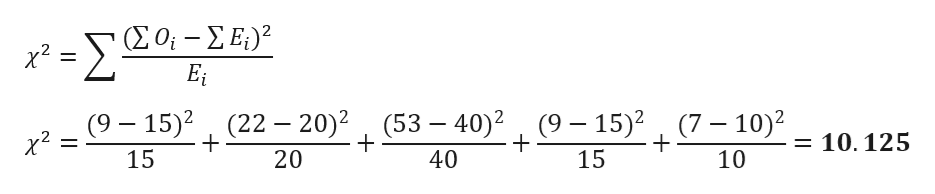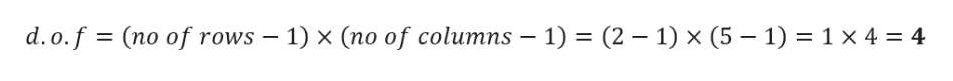# For the following observed and expected frequencies:Observed  9   22   53   9    7Expected  15  20  40  15  10a. Compute the value x square.b. How many degrees of freedom are there?c. Test the hypothesis that the distribution of the observed frequencies is as given by the expected frequencies. Use the a=0.01 level of significance.

Question
1 views

For the following observed and expected frequencies:

Observed  9   22   53   9    7

Expected  15  20  40  15  10

a. Compute the value x square.

b. How many degrees of freedom are there?

c. Test the hypothesis that the distribution of the observed frequencies is as given by the expected frequencies. Use the a=0.01 level of significance.

check_circle

Step 1

Given Data

Step 2

a)

The formula for the chi-square is given belowhelp_outlineImage Transcriptionclose-20-ΣΕ) (22 20)2 (53 40)2 (9-15)2 (7 10)2 (9-15)2 X x2 = 10.125 15 20 40 15 10 fullscreen
Step 3

b)

Degree of freedom is g...help_outlineImage Transcriptionclosed. o.f (no of rows 1) x (no of columns 1) (2 1) x (5 1) 1 x 4 4 fullscreen

### Want to see the full answer?

See Solution

#### Want to see this answer and more?

Solutions are written by subject experts who are available 24/7. Questions are typically answered within 1 hour.*

See Solution
*Response times may vary by subject and question.
Tagged in

### Statistics